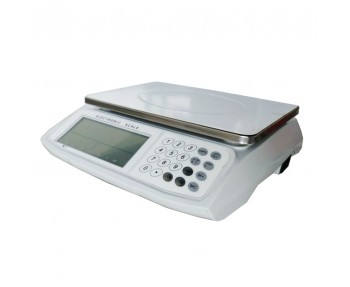A professional manufacture and sales of various electronic balances, moisture analyzers, viscometers and laboratory instruments.
weighinginstru@gmail.com

# Why Do We Need a Counting Scale?

2021/01/29

Why Do We Need a Counting Scale?

Imagine that an inventory check has to be done manually, and your store has thousands of parts to count. How many hours will it take to calculate everything? Also, it must be noted that stores may be closed while this work is being carried out, which may result in lost sales. Counting scales save time and money by eliminating the need for manual counting and speeding up the inventory counting process. It is said that once a counting scale is used, it will never return to manual counting.

Is that it? Should you purchase any counting scales to count? I wish it were as simple as that, but add some other elements. It is also important to choose the right scale for counting, as it is unfortunate to find that the scale you purchased is not counting correctly and is a waste of your time and money.

Did you know that items that look the same might have different weights? These items may be manufactured within production tolerances, different material stocks or even machining and operator tolerances. So, just because they look the same, they may not weigh the same. If you do not consider tolerances, errors can rapidly increase when counting bulk materials, leading to inaccurate counts.

So how can we avoid this? I've listed some points to consider when choosing the right scale.## Three steps for finding the optimal counting scale

### 1) Determine the average unit weight

Consider your application. If you are counting many different items with different weights, first determine the lightest item to count. This will determine the desired resolution from the scale. So, we have an item to count, take 10 of them, weigh them on a precise scale, and record the individual weights. Next, add the weights and divide by 10, and the result is the average average weight. This allows for variation in the tolerances of the parts. So, we now have the average weight of the minimum weight.

### 2) Determine the maximum weight

So we have a given average weight of objects, so we have to count. Are you talking about sending out the 100 pieces of production bagging? Or is there 10,000 units in your handbag and you're checking inventory? Either way, the maximum weight is essentially the average part weight times the number. If the average weight of my box of 10,000 screws is 2.5g, then the total weight is 10,000 x 2.5 = 25.0kg.

### 3) Choose a scale

Therefore, we know that the average weight and the maximum weight are 2.5g and 25.0kg respectively. Now let's determine the capacity of the scale.The average weight of our example is 2.5 grams. Counting scales must be able to measure and allow tolerance variations, so the average is usually divided by 10 or half of the average weight. In this case, we would reduce the average weight by half to 1.25 grams. This means I need to look for a scale with a weighing capacity of 1.25g or above or over 25kg. I know that in this case the CBC 32 has a resolution of 1G and a working capacity of 32kg, so it fits my needs.